# Experimental and finite element approach for finding sound absorption coefficient of bio-based foam

## L. Yuvaraj1, S. Jeyanthi2, Lenin Babu Mailan Chinnapandi3

1, 2, 3Vellore Institute of Technology, Chennai, India

2Corresponding author

Journal of Vibroengineering, Vol. 21, Issue 6, 2019, p. 1761-1771. https://doi.org/10.21595/jve.2019.20335
Received 25 October 2018; received in revised form 18 June 2019; accepted 30 June 2019; published 30 September 2019

Copyright © 2019 L. Yuvaraj, et al. This is an open access article distributed under the Creative Commons Attribution License, which permits unrestricted use, distribution, and reproduction in any medium, provided the original work is properly cited.
Views 1199
Abstract.

The enormous consumption of Polyurethane foam leads to severe environmental pollution and health hazards, so it is necessary to overcome this problem. This paper presents alternative and less hazardous foam that differs from traditional foams. A bio-based foam was developed either by using castor oil-based polyol or natural fibers as fillers. In the present study, rigid foam is synthesized by both castor polyol and luffa fiber, whereas for flexible foam, only luffa fiber is incorporated. Luffa fillers enhance the porosity of Polyurethane foam, which is the dominating factor influencing the value of the sound absorption coefficient. Both rigid and flexible foams were developed with 5, 10 and 15 percentages of filler loaded. The samples are tested experimentally using the two-microphone impedance tube method and the measured result was compared with the numerical result, which is predicted from COMSOL Multiphysics. The experimental results of flexible foam demonstrate good agreement with numerical results. The results indicate that the addition of Luffa fibers enhances the sound absorption performance of flexible foam and deterioration in the rigid foam because of the high viscosity of castor oil polyol.

Highlights
• Bio-based polyurethane foam developed with castor polyol and Luffa fiber for acoustic application.
• Incorporation of fibers enhances the sound absorption capability of Pu foam.
• Morphological study evident the presence of Luffa fiber in foam sample.
• Powder form of fiber can be used as filler rather than short fiber would be better for rigid foam.
• Flow resistivity of open cell foam increases with addition more fibers.

Keywords: polyurethane foam, luffa fiber, impedance tube, COMSOL Multiphysics, sound absorption coefficient.

#### 1. Introduction

A porous material is a combination of fluid phase and solid phase. The fluid phase percent is higher than that of the solid phase considered as a better absorbing material . The cell diameter is reduced by increasing the water content in the formulation that gives better acoustic damping efficiency at low frequencies. The better sound absorption coefficient of flexible foam obtained with the improved cellular structure by adding a high active catalyst .

Industrial polyurethane foam is produced by the reaction of di-isocyanate and polyol. The different proportions used for the reaction of di-isocyanate 2 (NCO) with the polyol (OH) give different forms of polyurethane foam. The proportion in which the NCO group percentage is more than that of the OH group leads to flexible foam (open cell foam). If the OH group percentage is higher than that of the NCO group, then it yields polyurethane foam for improving sound absorption properties . The natural fiber fillers improve the pore void percent, which is used in absorbing the acoustic pressure waves incident on them. Moreover, an increase in the thickness of the sample causes the enhancement in the sound absorption property . The formation of polyurethane foam is based on petrochemicals that have a serious effect on the human health and environment. To overcome this, natural fibers, which are renewable and bio-degradable, are used [4-6]. The increasing studies ensured environmental awareness which emphasized that the material should be produced in a version compatible with the environment. The replacement of synthetic fiber by natural fibers has been under serious consideration for environmental applications . There are various natural fibers like jute, rice hull, banana, so on. Among them, the Luffa fiber was chosen for this study. The Luffa fiber is a unique fiber which has the form of cellulose and hemicelluloses . The structure of Luffa fiber is unique, which has the form of a porous structure as a vascular structure. The experimental results showed that typical petroleum-based polyols replaced with soy-based polyol would make 75 % less environmental impact than petroleum-based ones. . The blend of a petrochemical polyol with 30 % of rapeseed oil and flex fiber reinforcement shows better influence on thermal and mechanical properties . Some of the materials used by researchers in their studies to achieve sound absorption were as follows: Kenaf , tea-leaf fibers , hemp fibers , jute fibers , cellulose fibers , agricultural waste , coconut fibers , textile fibers , cotton  and corn cob .

Luffa fibers were treated with sodium hydroxide solutions of 5 wt% for 4 h. Fibers were washed with distilled water to remove the excess of NaOH until neutral pH was achieved . Pre-treatment of Luffa fiber is a major step to avoid the moisture content and to make fiber stiff. From the perspective of acoustics, the pre-treatment process becomes necessary as it makes the pores acquire a homogeneous structure . Impedance tube technique is widely used for finding sound absorption properties of materials [5-6] where sound signals are generated from one end of the tube, and the samples to be conducted are placed at the other end according to the ASTM E-1050 . The sound absorption coefficient is calculated using two microphone techniques where the incident and reflected pressures are captured by the two microphones . The experimental data indicate that a 1 cm thick tea-leaf-fiber waste material with backing provides sound absorption, which is almost equivalent to that provided by six layers of woven textile cloth. The study shows that the use of tea leaf fiber of about 1cm thickness material shows equivalent sound absorption as compared with six layers of woven textile cloth .

FEM is a numerical method used to predict the acoustic behavior of layers or multilayer of porous materials, characterized by various shapes. COMSOL Multiphysics is the latest finite element (FEM) and partial differential equation (PDE) solution engine. In this paper, the Biot model is adapted to analyze the PU FOAM acoustic characteristics under different types of fiber incorporated. The polyurethane is a material which is a combination of fluid and solid structures. To proceed with this type of simulation, COMSOL Multiphysics is used to run the simulation . The Biot model is preferable to predict the acoustical behavior of materials. According to the Biot model for prediction, there are five important material parameters called Biot material parameters. The five parameters are the material porosity, airflow resistivity, tortuosity, thermal characteristic length, and viscous characteristic length. Different five test methods were developed to measure and predict these parameters .

The above literature confirms that the sound absorption analysis was carried out for various fibers and polyol, but Bio-based foam was developed from both natural based polyol, and short fiber was not reported. To fill this research gap, this study aims at measuring the sound absorption coefficient of Bio-Based polyurethane foam incorporated with LUFFA fibers in short forms with different percentages. The experimental investigation is conducted employing the impedance tube measurement technique. The finite elemental approach of Bio-Based polyurethane foam is made in COMSOL Multiphysics for numerical predictions. The results are compared, presented, and discussed in the following sections.

#### 2.1. Materials

In addition to the general application for noise control, polyurethane foam is used in various fields. However, due to some adverse effects on the environment and human health, the usage is limited. To improve the complete usage of polyurethane foam without compromising the effects of synthetic foam, a new approach is made in this study, Luffa cylindrica (LC) tropical plant belonging to the family of Cucurbitaceae. The advantage of Luffa is its vascular system with a fibrous arrangement. The other side of Luffa also can be used for improving the sound absorption coefficient. Luffa cylindrica is a naturally porous material with lots of small voids which benefits in the investigation of the sound absorption coefficient. The Luffa used in this work is chopped into short fibers of 2 mm long. The matrix of composite materials obtained in this work is Bio-Based polyurethane foam (open cell and closed cell). Formation of polyurethane foam constitutes polyol and isocyanate MDI (methylene diphenyl diisocyanate). The polyol is replaced from petrol-based to bio-based castor oil polyol and isocyanate is wannate 8018 which cannot be changed to bio-based one.

Table 1. Chemical formulation of polyurethane foam

 Name of chemical EMPEYOL WSF 100/S (pbw) Jagropol-400 (pbw) Polyol 100 100 DMCHA 1 1 Water 3.5 3.5 Surfactant 1.75 1.75 EMPEYONATE 0 115 WANNATE 8018 65 0 Density (kg/m3) 30 35

#### 2.2. Fabrication of natural polyol-based composite

In this work, before proceeding with the sample preparation to enhance interfacial adhesion, crystalline structure and minimizing moisture content, it becomes essential to treat the raw materials. Luffa fiber is treated with 5% of NaOH solution for 2-3 hours at a room temperature, and is cleaned with running water, and then dried for 2 days at a room temperature. We can see the apparent effect of alkali treatment on fibers from the formation of their structure. The effect of alkali treatment is shown in Fig. 1.

Fig. 1. (a) Luffa fiber; (b) after NaOH treatment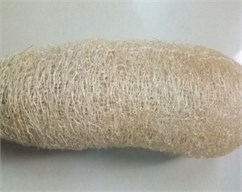a)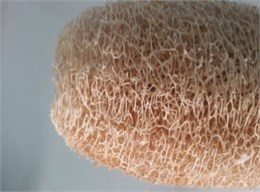b)

Luffa fiber chopped into 2 mm long portions and used in three different weight ratios of [5 wt%, 10 wt%, 15 wt%] in the composite has been chosen for this study which aims at measuring the effect caused on the sound absorption coefficient by reinforcing polyurethane foam with the chosen fiber. Polyurethane foam is made of constitutes of Polyol (EMPEYOL WSF 100/S) Isocyanate (WANNATE8018). The mixing ratio of 100:65 is used for open cell foam and Polyol (JAGROPOL 400) Isocyanate (EMPEYONATE), whereas it is 100:115 for closed cell foam. Both open cell and closed cell foams are incorporated with fibers to maintain bio-based and to improve the noise reduction property. A rectangular mold is used for making the polyurethane foam. Polyol and isocyanate with different wt% of the fiber is mixed in a beaker. A mechanical stirrer of 2000 rpm was used to mix the composition to obtain a homogeneous composition. After the mixing process is over, it freely is allowed to leave the foam at a room temperature for one hour. The circular samples, having diameters of 33 mm and 96 mm and the height of 30 mm, are cut out for impedance tube testing.

#### 2.3. Morphology of foam

Scanning Electron Microscopic (SEM) analysis is carried out using Zeiss, EV-18 model machine. The samples are coated with gold sputtering before scanning.

Fig. 2. (a) SEM image of LUFFA fiber, (b) presence of Luffa fiber in flexible foam, (c) void in rigid foam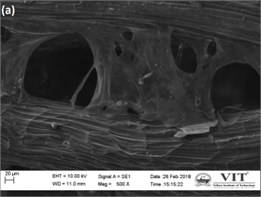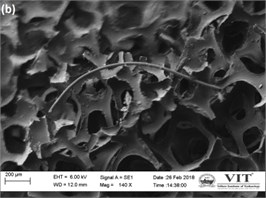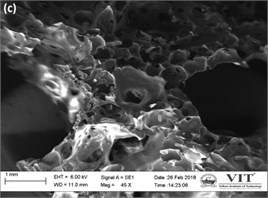#### 2.4. Measurements of acoustic properties

As per ASTM E1050-12, a two-microphone impedance tube is used to measure the sound absorption coefficient of the PU foam at a frequency between 220 and 4500 Hz, as shown in (Fig. 3). Sample cut into circular sections with diameters of 33 and 96 mm. Three samples for each category are used for this test. Intermittent random signal is generated from source channel DAQ connected to the power amplifier then to 16 Ω speakers at one end and two 1/2” MicrotechGefell microphone connected to M+P Vibpilot DAQ. Using a M+P spectrum analyzer, the time domain is extracted from the useful bandwidth of 6400 Hz with a frequency resolution of 0.5 Hz, which is imported to MATLAB®for calculating the transfer function between two microphones. The transfer function can be calculated using equations (1) and (2) from the data obtained from microphones 1 and 2.

Fig. 3. Impedance tube setup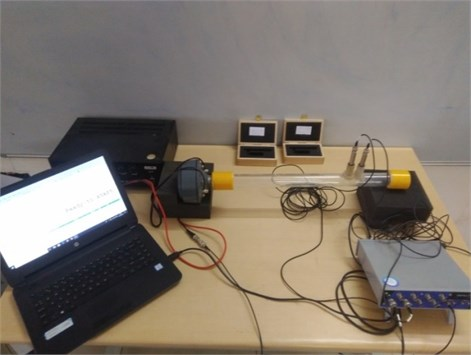Wave number:

(1)
$\left({K}_{0}\right)=\frac{2\pi f}{{C}_{0}},$

where $f$ is the frequency and ${c}_{0}$ is the speed of sound:

(2)
$\left({H}_{12}\right)=\frac{{p}_{2}}{{p}_{1}}=\frac{{e}^{j{k}_{0}{x}_{2}}+r{e}^{-j{k}_{0}{x}_{2}}}{{e}^{j{k}_{0}{x}_{1}}+r{e}^{-j{k}_{0}{x}_{1}}},$

where ${H}_{12}$ is the acoustic transfer function, ${p}_{1}$ and ${p}_{2}$ are the measured acoustic pressures of two microphones, and ${x}_{1}$ and ${x}_{2}$ are the distances between the reference plane (sample position of $x$ = 0) and the two microphones. The reflection and absorption coefficient obtained from the equations (3) and (4).

(3)
$R=\frac{{e}^{-jkS}-{H}_{12}}{{H}_{12}-{e}^{jkS}}×{e}^{2jk\left(l+S\right)},$
(4)
$\left(\alpha \right)=1-{\left|R\right|}^{2}.$

#### 3. Numerical model

This section presents two important formulations, and, if they are used, we can study and predict the acoustic behavior of poroelastic material. These formulations are developed by Atalla based on the classic formulation of Biot for porous rock. It is also called as the displacement-pressure (${u}^{s}$, P) formulation which comprises of 6 variables for 3D: 3 for solid phase and 3 for fluid phases, and the combined formulation that uses 4 space variables. The formulation of poroelastic material subjected to a single acoustic wave with angular frequency of $\omega$, incident on the porous material with elastic nature, and the wave equation in the solid strut and fluid are obtained from following equations.

For the solid phase:

(5)
$N{\nabla }^{2}{u}^{s}+\left(P-N\right)\nabla \nabla {u}^{s}+Q\nabla \left(\nabla {u}^{f}\right)+{\omega }^{2}\left({\stackrel{~}{\rho }}_{11}{u}^{s}+{\stackrel{~}{\rho }}_{12}{u}^{f}\right)=0.$

For the fluid phase:

(6)
$Q\nabla \left(\nabla {u}^{s}\right)-R\nabla \left(\nabla {u}^{s}\right)+\omega \left({\stackrel{~}{\rho }}_{12}{u}^{s}+{\stackrel{~}{\rho }}_{22}{u}^{f}\right)=0.$

The variables ${u}^{s}$ and ${u}^{f}$ are respectively the displacement vector in the structure and saturated fluid. The coefficients ${\stackrel{~}{\rho }}_{11}$, ${\stackrel{~}{\rho }}_{12}$ and ${\stackrel{~}{\rho }}_{22}$ are derived from the mass coupling factor, whereas for ${\rho }_{11}$, ${\rho }_{12}$ and ${\rho }_{22}$ are from viscous coupling factor $b$.

(7)
${\stackrel{~}{\rho }}_{11}={\rho }_{11}-j\frac{b}{\omega },$
(8)
${\stackrel{~}{\rho }}_{12}={\rho }_{12}+j\frac{b}{\omega },$
(9)
${\stackrel{~}{\rho }}_{22}={\rho }_{22}-j\frac{b}{\omega }.$

Mass coupling factor is obtained as follows:

(10)
${\rho }_{11}={\rho }_{1}-{\rho }_{22},$
(11)
${\rho }_{12}=-\varphi {\rho }_{0}\left({\alpha }_{\infty }-1\right),$
(12)
${\rho }_{22}={\varphi \rho }_{0}-{\rho }_{12}$

Viscous coupling parameter:

(13)
$b=\sigma {\varphi }^{2}\left[\sqrt{1+j\frac{4{\propto }_{\infty }^{2}\eta {\rho }_{air}\omega }{{\sigma }^{2}{\bigwedge }^{2}{\varphi }^{2}}}\right].$

Whereas $\varphi$ is the porosity, the elasticity coefficient $P$, $N$, $Q$, and $R$ are variables introduced by Biot. In case of a rigid frame, four elasticity coefficients are approximated by the following relations:

(14)
$P={\frac{4}{3}N+K}_{b}+\frac{{\left(1-\varphi \right)}^{2}}{\varphi }{K}_{f},$
(15)
$N=\frac{3{K}_{b}\left(1-2\nu \right)}{2\left(\nu +1\right)},$
(16)
$Q=\left(1-\varphi \right){K}_{f},$
(17)
$R=\varphi {K}_{f}.$

${K}_{b}$, ${K}_{f}$ are compressibility moduli of the solid and fluid regions corresponding to the dynamic tortuosity established for the JCA model :

(18)
${\rho }_{eff}=\frac{{\propto }_{\infty }{\rho }_{air}}{\varphi }\left[1+\frac{\sigma \varphi }{{j\omega \propto }_{\infty }{\rho }_{air}}\sqrt{1+j\frac{4{\propto }_{\infty }^{2}\eta {\rho }_{air}\omega }{{\sigma }^{2}{\bigwedge }^{2}{\varphi }^{2}}}\right],$
(19)
${K}_{eff}=\frac{\gamma {P}_{0}/\varphi }{\gamma -\left(\gamma -1\right)\left[1+\frac{\sigma \varphi }{{j{P}_{r}\omega \propto }_{\infty }{\rho }_{air}}\sqrt{1+j\frac{4{\propto }_{\infty }^{2}\eta {\rho }_{air}\omega {P}_{r}}{{\sigma }^{\text{'}2}{\Lambda }^{\text{'}2}{\varphi }^{2}}}\right]}.$

Characteristic impedance:

(20)
$\left({Z}_{P}\right)=\sqrt{{\rho }_{eff}*{K}_{eff}}.$

Wave number:

(21)
$\left(k\right)=\omega \sqrt{{\rho }_{eff}/{K}_{eff}}.$

Normalized surface impedance (${Z}_{sneq}$) of the equivalent fluid is obtained by:

(22)
${Z}_{sneq}=\frac{{Z}_{P}*\mathrm{c}\mathrm{o}\mathrm{t}\left(k*L\right)}{\varphi }*{Z}_{air}.$

Compute sound reflection ($R$) and absorption coefficient ($\alpha$):

(23)
$R=\frac{{Z}_{sneq}-1}{{Z}_{sneq}+1},$
(24)
$\left(\alpha \right)=1-{\left|R\right|}^{2},$

$\varphi$ is the porosity, ${\propto }_{\infty }$ is the tortuosity, is the flow resistivity, $\mathrm{\Lambda }$ is the viscous characteristics length, ${\mathrm{\Lambda }}^{\mathrm{\text{'}}}$ is the thermal characteristic length, ${\rho }_{air}$= 1.21 is the density of air in kg/m3, $\omega$ is the angular frequency, $\gamma$ = 1.14 is the specific heat ratio of air at the standard temperature, ${P}_{0}$ = 101320 is the atmospheric pressure in N/m2, $\eta$ = 1.84×10−5 is the viscosity of air in Ns/m2, $Pr$ = 0.702 is the Prandtl number of air, and L is the thickness of the porous material.

#### 3.1. Numerical prediction by COMSOL

A finite elemental procedure established to simulate the acoustical behavior of samples is used in the experimental approach. A porous material is a non-homogenous material which has a combination of the solid and fluid phases. Prediction of such material requires basic physical parameters. Different models are made to find the acoustical behavior of the material. Among those, the JCA model is preferable to predict the acoustical behavior of the materials. In such case, the JCA model needs for prediction five important parameters, the material has porosity, airflow resistivity, tortuosity, thermal characteristic length, and viscous characteristic length. All these five parameters predicted using inverse characterization technique by a Genetic algorithm are specified in Table 2 and 3, for flexible and rigid phases, respectively. The JCA model expressed effective density and bulk density in equations (18) and (19).

#### 3.2. Model Definition

In the modeled system, an incident sound field hits the porous foam layer at the angle of 0 degrees. The incident wave has the wave vector $k$. The model mentioned in this study consists of the height of 600 mm, the width of 100 mm, and the porous layer of 30 mm height as shown in Fig. 5. The incident field is modeled by applying a background pressure to the air domain. As for the boundary conditions of this model, it consists of pressure acoustics applied to the air domain as shown in the Fig. 5a). Sound hard boundary is applied on the top and bottom of the model to avoid the pressure field leaking outside. Poroelastic material is another boundary applied to the porous domain in which five Biot parameters are to be applied to the porous medium. The main advantage of COMSOL Multiphysics is that two different phases can couple by using the Multiphysics option from the software. A free triangular element with a mesh size of 0.16 times of the minimum wavelength is used in the analysis. Finally, the sound absorption coefficient, and the normal surface acoustic impedance, two important acoustic parameters of materials can be evaluated. The absorption coefficient results of the single case are shown in Fig. 5.

Fig. 5. Numerical model and result in COMSOL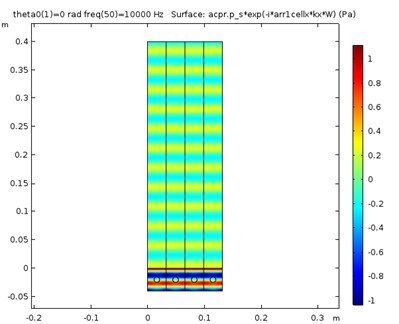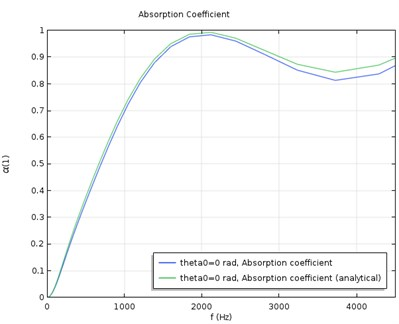#### 4. Results and discussion

The sound absorption coefficient of 30 mm thick foam with different density and different percentage of fiber loading with rigid backing is measured using the impedance tube and the same predicted by a JCA model using COMSOL.

#### 4.1. Effect of fiber on flexible porous material

The experimental sound absorption coefficient results of different fiber loading samples are shown in Fig. 6(a). The overall results show that the incorporation of fiber enhances the sound absorption, particularly with a lower frequency and leads to other significant benefits. As for the percentile, 0 % shows a smooth trend and average sound absorption, whereas the introduction of fiber increases the number of open cells in the flexible PU foam, which leads to effective absorption in a lower frequency. Meanwhile, the increases of fiber loading percentage provide the uniform distribution of open cell by forming a void. So, it acts as resonator showing a good result in a low frequency and oscillating trend in a higher frequency.

Moreover, the presence of fiber causes additional friction when sound propagates in it, and the same is confirmed with flow resistivity values depicted in Table 2. The results reveal that the improvement of sound absorption is attained by adding the fiber percentage of 5, 10 and 15 % when compared to a no-fiber sample. Among three fiber added samples, 15 % demonstrates good absorption, but in the mid-range frequency, there is a lag whereas 10 % is leading. From this data, we can clearly understand that the 10 % sample has a significant effect in the sound absorption coefficient. The validation results show good agreement with experimental and numerical values of below 1000 Hz beyond that the presence of void makes slight mismatch in the plot, but the relative error prediction of those values is acceptable within 10 %.

Table 2. Five parameters of flexible foam obtained from inverse characterization

 S. No $\varphi$ $\sigma$ (Ns/m4) ${\propto }_{\infty }$ $\mathrm{\Lambda }$ (µm) ${\mathrm{\Lambda }}^{\mathrm{\text{'}}}$ (µm) 1 0.91 12878 1.06 98 161 2 0.90 16558 1.01 98 167 3 0.90 53512 1.43 26 422 4 0.90 47734 1.49 19 76

Fig. 6. (a) SAC of flexible PU composite, (b) SAC of rigid PU foam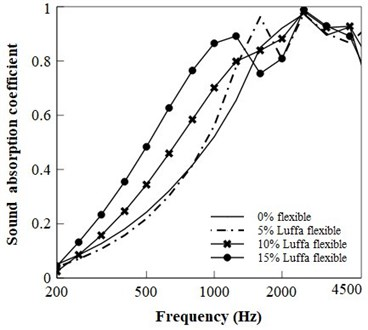a)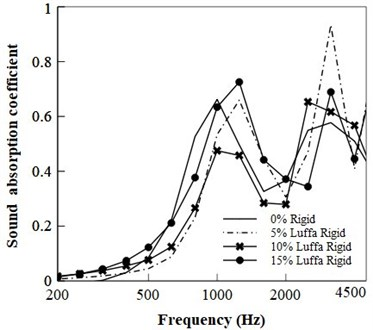b)

#### 4.2. Effect of fiber on rigid porous material

Fig. 6(b) shows the sound absorption coefficient values for all rigid samples across the measured range of all frequencies. Having the rigid nature, Pu foam possess less sound absorption, but it can be improved by fillers. Usually, rigid foams contain closed cells but the incorporation of luffa fiber disturbs the cell structure, which makes the close cell to open. Unfortunately, the fiber-loaded rigid foam samples fail to meet the expected results. This is mainly due to the viscous nature of castor polyol; when Luffa fiber stirred in polyol mixture has small bunches of fiber stuck together and settled randomly in the middle region of foams due to this, the closed cell structure spoiled it and led to the availability of voids of different diameter. This can be seen in SEM images in Fig. 2(c) as well as in the plot, fiber loaded sample shows vast deviation in the experimental and COMSOL results plot. Castor oil-based polyol foam may not be suitable for a short fiber or, by decreasing the viscosity, we can be able to use a short fiber as a filler in the rigid foam. Certainly, with the help of the properties get altered once, we can change the viscosity.

#### 4.3. Validation results

The predicted physical parameters values obtained by inverse characterization are depicted in Tables 1 and 2. The COMSOL sound absorption is plotted by giving the above physical parameter as the input one then it is compared to the experimental results. The validation graphs are shown in Fig. 7(a) for flexible foam and (b) for rigid foam. The validation demonstrates that predicted inputs make a good agreement for flexible foam, in the present case, sound absorption majorly depends on flow resistivity. For the case of rigid foam validation, as previously discussed, the introduction of fiber leads to foam collapse. So, the results do not show good agreements even from the predicted values; it is very difficult to understand these values. For instance, the tortuosity values are above 2 that means that the path is so tortuous, then the sound absorption should be good but here the absorption is poor. Powder form of fiber can be used to avoid this viscous problem for rigid foam samples in the future.

Fig. 7. (a) Validation of flexible PU composite, (b) validation of Rigid PU composite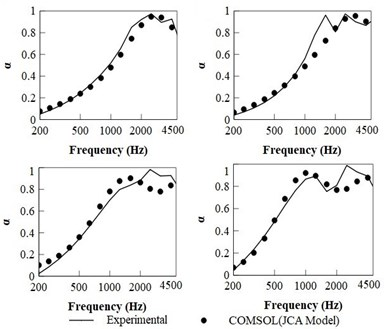a)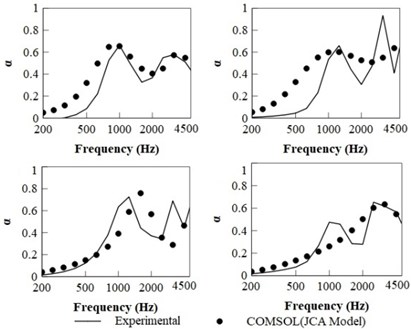b)

Table 3. Five parameters for rigid foam obtained from inverse characterization

 S. No $\varphi$ $\sigma$ (Ns/m4) ${\propto }_{\infty }$ $\mathrm{\Lambda }$ (µm) ${\mathrm{\Lambda }}^{\mathrm{\text{'}}}$ (µm) 1 0.50 20220 2.25 20 500 2 0.52 17395 2.49 26 500 3 0.32 37495 2.4 33 474 4 0.74 1000 1 250 240

#### 5. Conclusion

In conclusion, this study has been focused on developing a new bio-based material with eco-friendly and better acoustic properties. The rigid foam samples are developed with both natural polyol and fiber, in case of flexible foam, only natural fiber is used. Three different percentages of fiber are incorporated, and samples are tested in the impedance tube. Using COMSOL Multiphysics, the numerical sound absorption is predicted and compared to the experimental values. The validation results showed a good agreement for flexible samples and a mismatch for rigid ones.

Furthermore, the results indicate:

1. Incorporation of luffa fiber in flexible foam sample provides significant improvements in sound absorption of low frequency domain. As the percentage fiber increases, the peak sound absorption shifts towards the lower frequency region.

2. The physical parameters showed that the flow resistivity of flexible sample is increased because of the addition of natural fibers as well.

3. Rigid foam developed with a new attempt of natural polyol along with short fiber. Viscous nature of castor oil polyol arrests the fiber movement during the foaming process, which leads to the collapse of the foam.

4. Better sound absorption of rigid samples can be attained either by decreasing the viscosity, or a powder form of fiber can be used.

#### Acknowledgements

We would like to thank the Department of Science and Technology (DST-SERB; File No. ECR/2015/000111) for providing us the necessary facilities and funds for conducting this study.

1. Park Ju, Yang Hyun, Lee Hyeong Rae, Yu Cheng Bin Optimization of low frequency sound absorption by cell size control and multi-scale poroacoustics modeling. Journal of Sound and Vibration, 2017. [Publisher]
2. Gwon Jae Gyoung, Kim Seok Kyeong, Kim Jung Hyeun Sound absorption behaviour of flexible polyurethane foams with distinct cellular structures. Materials and Design, Vol. 89, Issue 5, 2016, p. 448-454. [Publisher]
3. Pinto Moises L. Formulation, preparation, and characterization of polyurethane foams. Journal of Chemical Education, Vol. 87, Issue 2, 2010, p. 1749-16. [Publisher]
4. Ekici Bulent, Kentli Aykut, Kucuk Haluk Improving sound absorption property of polyurethane foams by adding tea-leaffibers. Archives of Acoustics, Vol. 37, Issue 4, 2012. [Search CrossRef]
5. Ibrahim M. A., Melik R. W. Optimized sound absorption of rigid polyurethane foam. Archives of Acoustics, Vol. 28, Issue 4, 2003, p. 305-312. [Search CrossRef]
6. Mohanta N., Achary S. K. Fiber surface treatment: its effect on structural, thermal, and mechanical properties of Luffa cylindrica fiber and its composite. Journal of Composite Materials, 2015. [Publisher]
7. Suqin Tan, et al. Rigid polyurethane foams from a soybean oil-based polyol. Polymer, Vol. 52, 2011, p. 2840-2846. [Publisher]
8. Maria Kuranska, et al. Porous polyurethane composites with natural fibres. Composites Science and Technology, Vol. 72, 2012, p. 299-304. [Publisher]
9. Nar M., Webber III C., D’Souza N. A. Rigid polyurethane and kenaf core composite foams. Polymer Engineering Science, Vol. 55, 2015, p. 132-144. [Publisher]
10. Ekici B., Kentli A., Kucuk H. Improving sound absorption property of polyurethane foams by adding tealeaf fibers. Archives of Acoustic, Vol. 37, Issue 4, 2012, p. 515-520. [Publisher]
11. Glé P., Gourdon E., Arnaud L. Acoustical properties of materials made of vegetable particles with several scales of porosity. Applied Acoustic, Vol. 72, 2011, p. 249-259. [Publisher]
12. Fatima S., Mohanty A. R. Acoustical and fire-retardant properties of jute composite materials. Applied Acoustic, Vol. 72, 2011, p. 108-114. [Publisher]
13. Li Y., Ren H. F., Ragauskas A. J. Rigid polyurethane foam reinforced with cellulose whiskers: Synthesis and characterization. Nano-Micro Letter, Vol. 2, Issue 2, 2010, p. 89-94. [Publisher]
14. Binici H., Eken M., Dolaz M., Aksogan O., Kara M. Environmentally friendly thermal insulation material from sunflower stalk, textile waste and stubble fibres. Construction of Building Materials, Vol. 51, 2014, p. 24-33. [Publisher]
15. Shan C. W., Idris M. I., Ghazali M. I. Study of flexible polyurethane foams reinforced with coir fibres and tyre particles. International Journal of Applied Physics and Maths, Vol. 2, Issue 2, 2012, p. 123-130. [Publisher]
16. Curtu I., Stanciu M. D., Cosereanu C., Vasile O. Assessment of acoustic properties of biodegradable composite materials with textile inserts. Materiale Plastice, Vol. 49, 2012, p. 68-72. [Search CrossRef]
17. Binici H., Gemci R., Kucukonder A., Solak H. H. Investigating sound insulation, thermal conductivity and radioactivity of chipboards produced with cotton waste, fly ash and barite. Construction and Building Materials, Vol. 30, 2012, p. 826-832. [Publisher]
18. Paiva A., Pereira S., Sá A., Cruz D., Varum H., Pinto J. Contribution to thermal insulation performance characterization of corn cob particleboards. Energy Buildings, Vol. 45, 2012, p. 274-279. [Publisher]
19. Tiuca Ancuţa-Elena, Vermeşana Horaţiu Improved sound absorption properties of polyurethane foam mixed with textile waste. Energy Procedia, Vol. 85, 2016, p. 559-565. [Publisher]
20. Silva André Leandroda, Silva Lucas Renan Rochada, Camargo Isabellede Andrade Cardanol-based thermoset plastic reinforced by sponge gourd fibers (Luffacylindrica). Polímeros, Vol. 26, Issue 1, 2016. [Publisher]
21. Yuvaraj L., Jeyanthi S. Numerical and Experimental characterization of acoustic porous material: review. International Journal of Mechanical Engineering and Technology, Vol. 8, Issue 8, 2017, p. 919-930. [Search CrossRef]

#### Cited By

 Materials Today: Proceedings Yuvaraj L, K.P. Prashanth, B.K. Venkatesha, S. Sanman, N. Venkatesh 2022 Journal of Porous Materials Vinoth Kumar Selvaraj, Jeyanthi Subramanian 2022 International Journal for Simulation and Multidisciplinary Design Optimization L. Yuvaraj, S. Jeyanthi, Lenin Babu Mailan Chinnapandi, Elammaran Jayamani, Nadhir Lebaal, Subramanian Jeyanthi, Jebaseelan Davidson, M.C. Leninbabu 2021 IOP Conference Series: Materials Science and Engineering M Gokul, T Gowtham, S Jeyanthi, M C Lenin babu, Elammaran jayamani 2021 INTERNATIONAL CONFERENCE ON SCIENCE AND APPLIED SCIENCE (ICSAS2020) Romy Dyah Rahmawati, Mohammad Masykuri, Yulia Lanti Retno Dewi 2020